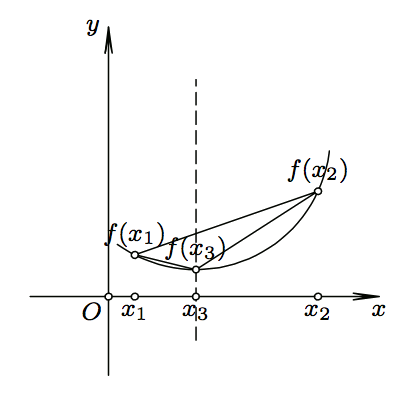# Convex Functions

To prove some of the fundamental results we will need to use convexity of certain functions. Proofs of the theorems of Young, Minkowski, and Holder will require us to use very basic facts -- you should be fine if you just read the definition of convexity and the example in which some famous convex functions are listed. However, the section on Karamata’s inequality will require some deeper knowledge which you can find here.

Definition (Convexity)

The function $$f:[a,b] \rightarrow \mathbb R$$ is convex if for any $$x_1,x_2\in[a,b]$$ and any $$\lambda \in (0,1)$$ the following inequality holds: \begin{eqnarray*} f(\lambda x_1+(1-\lambda)x_2)\leq \lambda f(x_1)+(1-\lambda)f(x_2). \quad\quad\quad\quad\quad (1) \end{eqnarray*} Function is called concave if $$-f$$ is convex. If the inequality in (1) is strict then the function is called strictly convex.Now we will give a geometrical interpretation of convexity. Take any $$x_3\in(x_1,x_2)$$. There is $$\lambda\in(0,1)$$ such that $$x_3=\lambda x_1+(1-\lambda) x_2$$. Let’s paint in green the line passing through $$x_3$$ and parallel to the $$y$$ axis. Let’s paint in red the chord connecting the points $$(x_1,f(x_1))$$ and $$(x_2, f(x_2))$$. Assume that the green line and the red chord intersect at the yellow point. The $$y$$ coordinate (also called the height) of the yellow point is: $\lambda f(x_1)+(1-\lambda)f(x_2).$ The inequality (1) means exactly that the the green line intersects the graph of a function below the red chord. If $$f$$ is strictly convex then the equality can hold in (1) if and only if $$x_1=x_2$$.

Example

The following functions are convex: $$e^x$$, $$x^p$$ (for $$p\geq 1$$, $$x> 0$$), $$\frac1x$$ ($$x\neq 0$$), while the functions $$\log x$$ ($$x> 0$$), $$\sin x$$ ($$0\leq x\leq \pi$$) , $$\cos x$$ ($$-\pi/2\leq x\leq \pi/2$$) are concave.

All functions mentioned in the previous example are elementary functions, and proving the convexity/concavity for them would require us to go to the very basics of their foundation, and we will not do that. In many of the examples and problems respective functions are slight modifications of elementary functions. Their convexity (or concavity) is something we don’t have to verify. However, we will develop some criteria for verifying the convexity of more complex combinations of functions.

Let us take another look at our picture above and compare the slopes of the three drawn lines. The line connecting $$(x_1, f(x_1))$$ with $$(x_3, f(x_3))$$ has the smallest slope, while the line connecting $$(x_3, f(x_3))$$ with $$(x_2, f(x_2))$$ has the largest slope. In the following theorem we will state and prove that the convex function has always an increasing slope.

Theorem 1.

Let $$f:[a,b]\rightarrow \mathbb R$$ be a convex function and $$a\leq x_1< x_3< x_2\leq b$$. Then \begin{eqnarray} \frac{f(x_3)-f(x_1)}{x_3-x_1}\leq \frac{f(x_2)-f(x_1)}{x_2-x_1} \leq \frac{f(x_2)-f(x_3)} {x_2-x_3}. \quad\quad\quad\quad\quad (2) \end{eqnarray}

The rest of this chapter is using some of the properties of limits, continuity and differentiability. If you are not familiar with basic calculus, you may skip that part, and you will be able to understand most of what follows. Theorem 3 is a tool for verifying the convexity for differentiable functions that we mentioned before. The theorem 3 will be used it in the proof of Karamata’s inequality.

Theorem 2

If $$f: (a,b)\rightarrow \mathbb R$$ is a convex function, then $$f$$ is continuous and at every point $$x\in(a,b)$$ it has both left and right derivative $$f^{\prime}_-(x)$$ and $$f^{\prime}_+(x)$$. Both $$f^{\prime}_-$$ and $$f^{\prime}_+$$ are increasing functions on $$(a,b)$$ and $$f^{\prime}_-(x)\leq f^{\prime}_+(x)$$.

Theorem 3

If $$f: (a,b)\rightarrow \mathbb R$$ is a twice differentiable function. Then $$f$$ is convex on $$(a,b)$$ if and only if $$f^{\prime\prime}(x)\geq 0$$ for every $$x\in(a,b)$$. Moreover, if $$f^{\prime\prime}(x)> 0$$ then $$f$$ is strictly convex.

2005-2021 IMOmath.com | imomath"at"gmail.com | Math rendered by MathJax